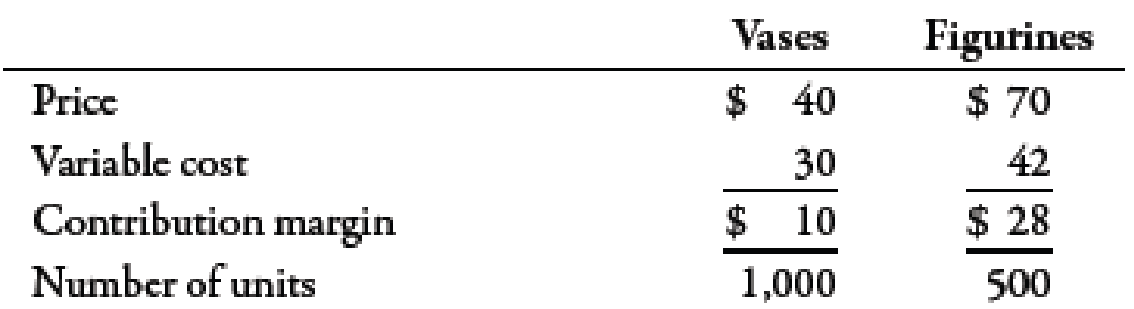Chapter 7, Problem 49E### Managerial Accounting: The Corners...

7th Edition
Maryanne M. Mowen + 2 others
ISBN: 9781337115773

#### Solutions

Chapter
Section### Managerial Accounting: The Corners...

7th Edition
Maryanne M. Mowen + 2 others
ISBN: 9781337115773
Textbook Problem
5 views

# Parker Pottery produces a line of vases and a line of ceramic figurines. Each line uses the same equipment and labor; hence, there are no traceable fixed costs. Common fixed cost equals $30,000. Parker’s accountant has begun to assess the profitability of the two lines and has gathered the following data for last year:Required: 1. Compute the number of vases and the number of figurines that must be sold for the company to break even. 2. Parker Pottery is considering upgrading its factory to improve the quality of its products. The upgrade will add$5,260 per year to total fixed cost. If the upgrade is successful, the projected sales of vases will be 1,500, and figurine sales will increase to 1,000 units. What is the new break-even point in units for each of the products?

1.

To determine

Calculate the break-even quantity for vases and figurines.

Explanation

The package of vases and figurines is given below:

 Product Price ($) A Unit Variable Cost ($) B Unit Contribution Margin ($) C (A−B) Sales Mix D Package Unit Contribution Margin ($) (C×D) Vases 40 30 10 2 20 Figurines 70 42 28 1 28 Total 48

Table (1)

Use the following formula to calculate break-even quantity:

Break-even quantity=Total fixed costContribution margin

Substitute $30,000 for total fixed cost and$48 for contribution margin in the above formula.

Break-even quantity=$30,000$48=625units

Therefore, break-even quantity is 625 units.

Use the following formula to calculate break-even quantity for vases:

Break-even quantity=Number of units×Sales mix

Substitute 625 units for number of units and 21 for sales mix in the above formula.

Break-even quantity=625×2=1,250units

Therefore, the break-even quantity for Vases is 1,250 units

2.

To determine

Calulate break-even quantity for vases and figurines after upgrading the factory.

### Still sussing out bartleby?

Check out a sample textbook solution.

See a sample solution

#### The Solution to Your Study Problems

Bartleby provides explanations to thousands of textbook problems written by our experts, many with advanced degrees!

Get Started